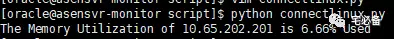# 使用paramiko获取linux系统内存使用率

Linux系统为 Centos 6.8

Python环境为 Python 3.6

## 获取内存使用率程序

``````def getlinuxmem(ssh):
result=[]
stdin,stdout,stderr=ssh.exec_command('free -m |awk \' NR==2 {print ((\$3 - \$6 - \$7)/\$2)*100}\'')
if len(err) != 0:
print (err)
return False
else:
result= stdout_content
try:
if  len(result) !=0:
return round(float(result.strip()),2)
else:
print ('There is something wrong when execute free command')
except Exception as e:
print (e)
``````

## 验证结果https://github.com/bsbforever/wechat_oms